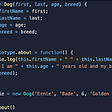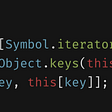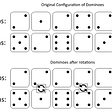# How integers are stored in memory using two’s complement.

According to Wikipedia “An integer (from the Latin integer meaning “whole”) is colloquially defined as a number that can be written without a fractional component.

That means that numbers such as “21”, “189” or “-1824” are integers, while “8.5” , “13 1/2” or “√4” are not. The number “0” (zero) is also an integer.

As we know, computers, in order to understand and store data (such as integer numbers) have to encode such data as binary numbers, which are numbers expressed in the base-2 numeral system. This system is known as base-2 because it only uses two symbols (typically 0 and 1), instead of the 10 symbols used by the decimal system.

A binary number is a sequence of bits. The specific position of the 0’s and 1’s within the sequence of bits creates the representation of the number’s value.

## Bit what?

Now, a bit is the most basic unit of the computer memory. Going back to Wikipedia: “The bit is a basic unit of information in computing and digital communications. The name is a portmanteau of binary digit. The bit represents a logical state with one of two possible values. These values are most commonly represented as either “1”or”0", but other representations such as true/false, yes/no, +/−, or on/off are common.

Physically, there are several ways to store a bit, but ultimately, it depends on the physical device capability to differentiate between two states. Whatever the physical implementation, the important thing to know about a bit is that, like a switch, it can only take one of two values: it is either “on” or “off”.

Eight bits placed together are called a byte. In a 32-bit or 64-bit computer, an integer value is stored in a collection of 4 bytes, or 32 bits.

So, if we consider the integer value “50”, the computer will store it in memory within a collection of bits of value 0 or 1.

The image of the Windows calculator above is actually filtering out all the leading 0’s in the binary value. The actual value stored would be something like this:

# 00000000 00000000 00000000 00110010

But what happens when representing negative integers such as -50?

Well, negative and positive are the two possible states of an integer value. In binary, each state can be represented by what is called the Most Significant Bit (MSB). The MSB will be the value of the first bit to the left of the binary number. If the integer number is positive, the MSB will have a value of 0, but if the number is negative, the bit is 1.

So -50 will be:

# 10000000 00000000 00000000 00110010

The problem is that using MSB to represent negative numbers has some important disadvantages, starting with the fact that reserving one bit to represent the negative/positive value of the number reduces the amount of available numbers by one half.

At the same time, using MSB would allow us to represent a value that actually doesn’t exist on decimal numeration such as “-0”. Like this:

# 10000000 00000000 00000000 00000000

## Enters one’s complement:

A possible work around to the reduction of the range of numbers is to utilize the method called one’s complement.

This method represents negative numbers by inverting the values of bits of the number magnitude. In plain English this means to flip all the bit values within the binary number to its opposite. A 1 is placed whenever there was a 0 and vice versa. The signed binary number obtained from the inversion is called complement.

So, again, 50 and -50:

00000000 00000000 00000000 00110010
11111111 11111111 11111111 11001101

While this significantly increases the amount of available numbers, it still has the issue of allowing the representation of a negative 0, which again, doesn’t actually exists:

11111111 11111111 11111111 11111111 (-0???).

## What about two’s complement then?

Wikipedia (again): “Compared to other systems for representing signed numbers (e.g., ones’ complement), two’s complement has the advantage that the fundamental arithmetic operations of addition, subtraction, and multiplication are identical to those for unsigned binary numbers (as long as the inputs are represented in the same number of bits as the output, and any overflow beyond those bits is discarded from the result). This property makes the system simpler to implement, especially for higher-precision arithmetic. Unlike ones’ complement systems, two’s complement has no representation for negative zero, and thus does not suffer from its associated difficulties.

## But how?

In a nutshell:

1- Find the binary equivalent for any given decimal.
2- Find the one’s complement of the binary by inverting 0's and 1’s.
3- Add 1 to the one’s complement.

Let’s start again with the integer 50 in binary:

00000000 00000000 00000000 00110010

Now let’s calculate -50 with the one’s complement method:

11111111 11111111 11111111 11001101

Finally we are going to add one (+1 in binary, of course) to -50.

11111111 11111111 11111111 11001101 +

00000000 00000000 00000000 00000001 (this is the binary representation of the decimal integer “1”).

Result:

11111111 11111111 11111111 11001101

The fact that the first bit on the left is a “1” indicates that the number is negative.

The range of integers obtained from using the two’s complement method is the same as the obtained from the one’s complement, but by using the two’s method a negative zero value will not be represented, as “1” it is always added to the complement of zero.

Decimal “0” — 00000000 00000000 00000000 00000000

“-0” (not a thing) — 11111111 11111111 11111111 11111111

addition of 1 -00000000 00000000 00000000 00000001

Result: 00000000 00000000 00000000 00000000 (actually 0!).

So, that are the reasons why computers use two’s complements to deal with negative values.

Hope you enjoyed!

--

--

--

## More from Flavio Orlando

Love podcasts or audiobooks? Learn on the go with our new app.

## JS Interview Help: Prototype, Class(ES6), IIFE, Scope, Closures, Module Pattern## Host Gatsby.js site on amazon s3 with AWS CodePipeline## Everything you need to know about JavaScript symbols## Next.js Typescript Netlify Forms — The no-redirect version## 3 Ways to include component in angular application## Speech text Reader with Javascript## React state management patterns in 2019## Challenge 2: Wireframes## [LeetCode][C++] #1007. Minimum Domino Rotations For Equal Row## BlogPost#1 — What is Truth?## 05 Appreciate what you have